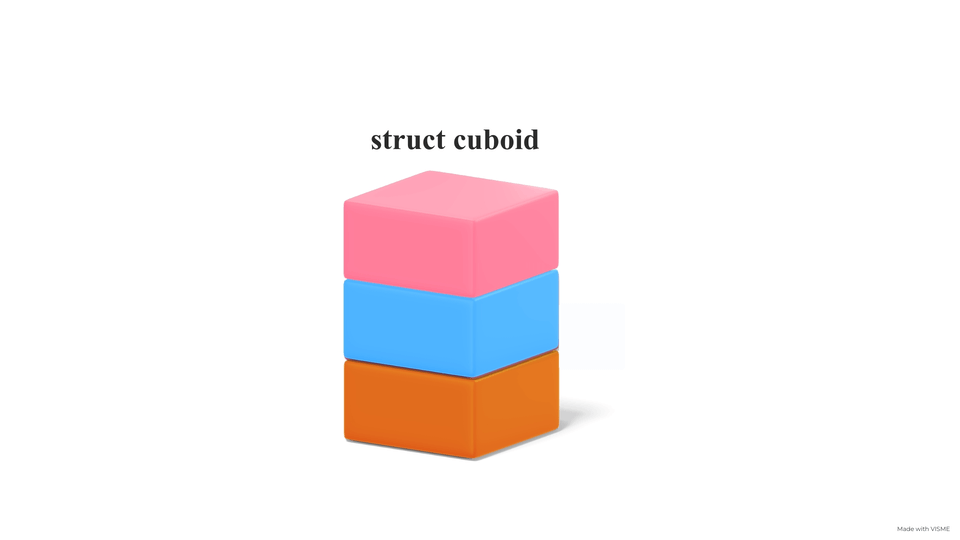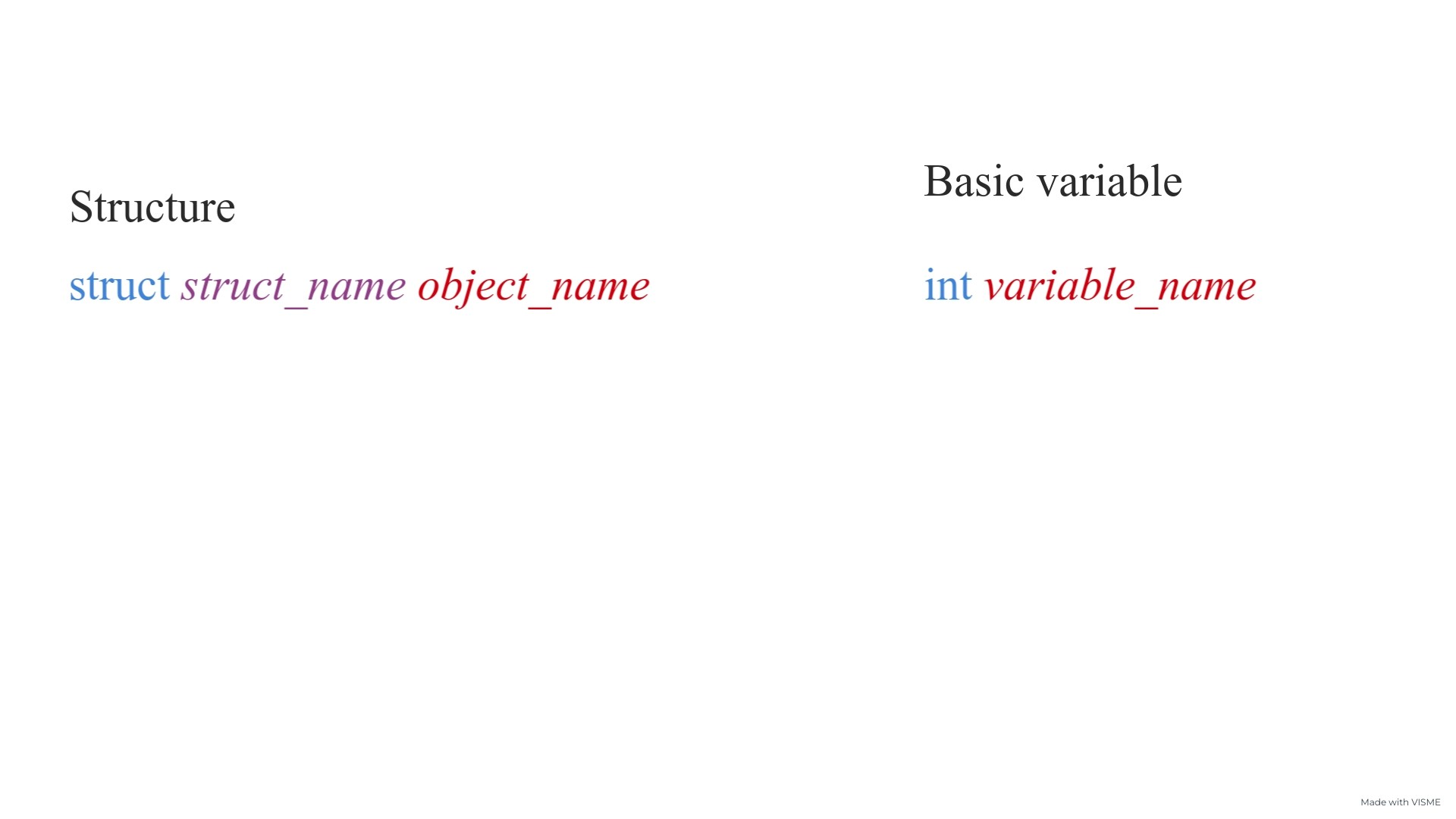# Structures in CCreated April 14, 2022

While coding in C, you will come across many data types like - char, int, float, double, void, and so on... These data types are called basic data types. There's another group of data types in c called - derived data types. These data types are derived from the basic data types. Thats why the name "derived" data types! Arrays, pointers, enum, etc. are some examples of this class of data types.

Structures also come under derived data types in C. In this article, I'm gonna tell you about Structures and how to use them in C Language. Let's get started!

## Structure

Structure (struct) is a user defined data type in which you can include any number of variables of any kind of data type. All these variables are said to be member variables of the structure. It's implementation in C is as follows -

### Syntax

``````struct cuboid{
int length=1;
int width=2;
int height=3;
};
``````

Here as we can see - there are 3 fields(or member variables) inside the structure called "cubiod". They're - length, width, height. Now you can put a default value for these variables like I've done in the above code, or you can define their values later on in the code once you define an object of this struct type.### Defining the object

You can easily define an object of the struct type like this -

``````#include <stdio.h>

struct cuboid{
int length=1;
int width=2;
int height=3;
};

int main(){
struct cuboid cuboidObject;
}
``````

So here I have just defined an object of struct type "cuboid" and the name of the object is "cuboidObject". The only difference between defining an int variable and struct datatype object is that you have to write two keywords for defining struct object whereas only one keyword has to be used for basic datatype variables.### Accessing the member variables

Now that we know how to define a structure and an instance of it(object), let's see how to access an object's member variables.

It can be easily done by using this syntax -

<object_name>. Example:

``````int main(){
struct cuboid cuboidObject;
printf("CUBOID\nLength: %d\nWidth: %d\nHeight: %d\n",cuboidObject.length, cuboidObject.width, cuboidObject.height);
}
``````

The above code is accessing the member variables of the object named "cuboidObject" of type "struct cuboid". The member variables are -

1. Length,
2. Width,
3. Height

And so they've accessed in the printf statement using the following syntax:

1. cuboidObject.length
2. cuboidObject.width
3. cuboidObject.heigth

Now, you can also modify the value of these member variables by simply setting them equal to some other value. Like -

``````int main(){
struct cuboid cuboidObject;
printf("nHeight: %d\n", cuboidObject.height);
cuboidObject.height = 50;
printf("nHeight: %d\n", cuboidObject.height);
}
``````

Both the print statements will print different results as the value for height of the object has been changed from 3 to 50.

### Nested structures

``````struct person{
char name;
int age;
};

struct student{
struct person p; //nested inner struct
char reg_no;
};
``````

Here, there are two structures - "person" and "student". Person structure has two variables- name and age. Student structure has 2 variables- a "person" structure object and reg_no. So, the idea of some structure object to be a member variable of another structure can be called "Nested structures".

## This is it!

Hope you liked reading this article and gained something from it. For more such articles on Data Structures and Algorithms, you can visit my blog here.